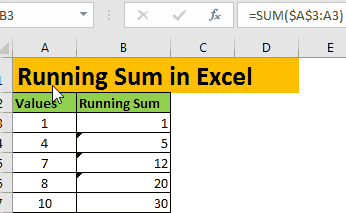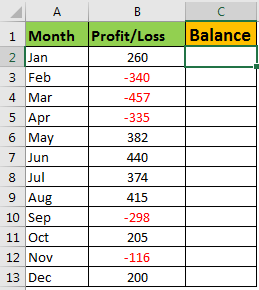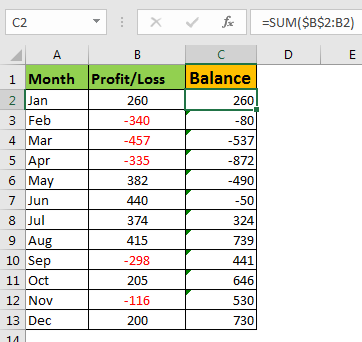# How to Calculate Running Balance in Excel

Earlier, we learned about how to do a running count of items occurrence in a list. In this article, we will learn how to calculate the running balance in Excel sheet. It is also called the running total. In other words, we will do a running sum. In running total, the last value is summed up with all values above it. See the gif below.Generic Formula for Running Count of Occurrence

Expanding reference: it is a reference that expands when copied down. In this reference, the first part is locked or says have absolute reference and second is relative reference. For example, \$A\$1:A1. To understand more, you can read the article linked above.

Running Sum Example

Let’s say you invest in the share market. You track your every month's profit and loss in an excel sheet. In column A, you have month's names. In column B, you have loss or profit made in the respective month. Profits are positive numbers, and negatives are losses. In column C, you won't have balance since you started it. In Jan, it will be the only Jan's balance. In Feb, it will be jan+feb. In Mar, it will be jan+feb+mar, and so on.Write this running sum formula in C2 and drag it down.

=SUM(\$B\$2:B2)

The result will look like this.Now, you can see how much you were at loss or profit at the end of each month since you started. You can plot a line graph to visualize your growth over the period.How It Works:

The trick is the expanding ranges. As we know, SUM function returns the sum of a given values in a range. Now in first cell C2, we have range \$B\$2:B2. Since this range has only one cell, we get the same value. In second cell C3. The range changes to \$B\$2:B3. We are summing now two cells B2 and B3. We get the sum of the two numbers above. This happens till C13. In C13 we will have range \$B\$2:B13 for sum. So yeah, we learned how to calculate running balance in Excel. If you have any question regarding this article or any other feature of excel 2016, 2013 and 2010, feel free to ask in the comments section below.

Related Articles:

How to Count Cells that contain specific text in Excel

Get the COUNTIFS Two Criteria Match in Excel

Get the COUNTIFS With OR For Multiple Criteria in Excel

How to use the COUNTIF function in Excel

Popular Articles :

50 Excel Shortcut to Increase Your Productivity : Get faster at your task. These 50 shortcuts will make you work even faster on Excel.

How to use the VLOOKUP Function in Excel : This is one of the most used and popular functions of excel that is used to lookup value from different ranges and sheets.

How to use the COUNTIF function in Excel : Count values with conditions using this amazing function. You don't need to filter your data to count specific values. Countif function is essential to prepare your dashboard.

How to use the SUMIF Function in Excel : This is another dashboard essential function. This helps you sum up values on specific conditions.

Terms and Conditions of use

The applications/code on this site are distributed as is and without warranties or liability. In no event shall the owner of the copyrights, or the authors of the applications/code be liable for any loss of profit, any problems or any damage resulting from the use or evaluation of the applications/code.